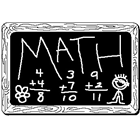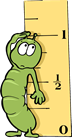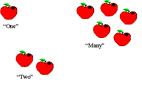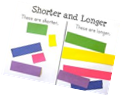# PreKindergarten Math

•PreKindergarten Overview

Mathematics

Students enrolled in a prekindergarten math class in Wicomico County Public Schools can expect to learn how to do the following:

Numbers and Counting

Know number names and the count sequence

• Build concept of number
• Show an understanding of quantity
• Construct relationships based on quantity
• Use classroom experiences to indicate same, more, or less
• Count and discuss quantity
• Use concrete materials to build sets 0 to 10
• Match a numeral to a set 0 to 10
•  Count to 20
• Identify written numerals to 10

Operations and Algebraic Thinking

Understand addition as putting together and subtraction as taking apart

• Represent simple addition and subtraction problems with objects
• Decompose a quantity to 10 into pairs in more than one way

Measurement and DataRecognize and use measurement attributes

• Demonstrate an understanding of: bigger, smaller, longer, shorter, lighter, heavier, shorter, taller
• Compare and describe objects according to a single attribute

Geometry

Recognize and use the attributes of plane and solid geometric figures

• Sort objects by one attribute such as: shape, color, size, weight, or length
• Name the attributes of plane figures such as: shape, color, size, number of sides
• Match triangles, circles, squares, rectangles, hexagons, and octagons of various sizes
• Identify shapes in the environment

Math Questioning

Numbers and Counting• How far can you count?
• Is your set the same size as any other sets? How do you know?
• Do you have more or less than 5 items? How do you know?
• How many would you have if I gave you one more?
• How many would you have if I took one away?
• How does your set compare to a set of 3 objects? Are there more or less?
• If I gave you two sets of connecting links, how many links would you have altogether?
• How do you know which set has more?
• How do you know which set has less?
• Is there a big difference or a small difference between the two sets?
• How many more objects does the larger set contain?
• How could the sets be changed so they have the same amount?
• What is the total number of cubes in both of the sets?

Measurement and Data• How do you know your object is smaller than this one?
• Can you find an object that is bigger than the one you chose?
• Can you find an object that is smaller than the one you chose? How do you know the object is smaller?
• Which object is the longest (shortest)? Show me how we can check that to be sure.
• What is longer (shorter) than the objects you have chosen?
• What object is heavier than this one? How do you know it is heavier?

Geometry

• Describe the objects. How will you sort them?
• Why did you decide to put the _____ there?
• Why are these objects sorted this way?
• Where would this object belong? How do you know?
• Can you think of anything else that could belong in this group?
• Why can't the _____ go in this group?
• Is there anything else that could go inside this group?
• Can you think of a different way to sort these objects?
• How many ways can you sort these objects?